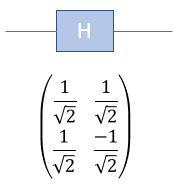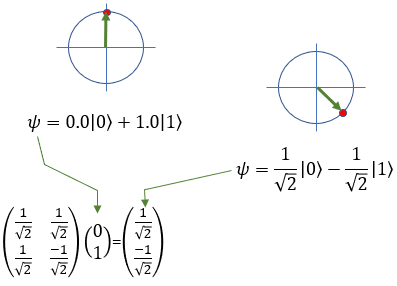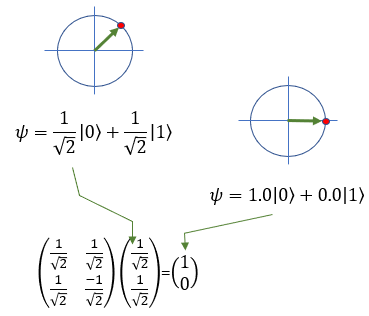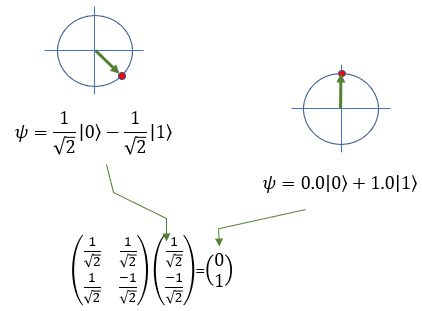Quantum Computing Quamtum Gate - H (Hadamard)   Hadamard Gate is one of the most famous Gate in Quantum Computing. It takes a state of a Qbit and produce the equal mixture of |0> and |1>.  It is represented as follows.As I said, it would always be confusing if you try to understand the quamtum operation verbally. Eventually you would need to turn to mathematics to have clearer and long lasting understanding.   First, let's take an example where a Qbit with the probability of 100% for |0> and 0% of |1> go through H(Hadamard) gate. This procedure results in a Qbit with the probability of 50% for |0> and 50% for |1>. It can be represented mathematically as shown below. (If you are not familiar with mathematical representation, try reading Qbit page)Now, let's take another example where a Qbit with the probability of 0% for |0> and 100% of |1> go through H(Hadamard) gate. This procedure results in a Qbit with the probability of 50% for |0> and 50% for |1>. It can be represented mathematically as shown below. (If you are not familiar with mathematical representation, try reading Qbit page)How about applying H gate to the superpositioned state. Following is an example. Application of H Gate to the superpositioned states of |0> gives the original state, meaning |0> - [H] - [H] gives |0>.Let's take a look at another example. Application of H Gate to the superpositioned states of |1> gives the original state, meaning |1> - [H] - [H] gives |1>.